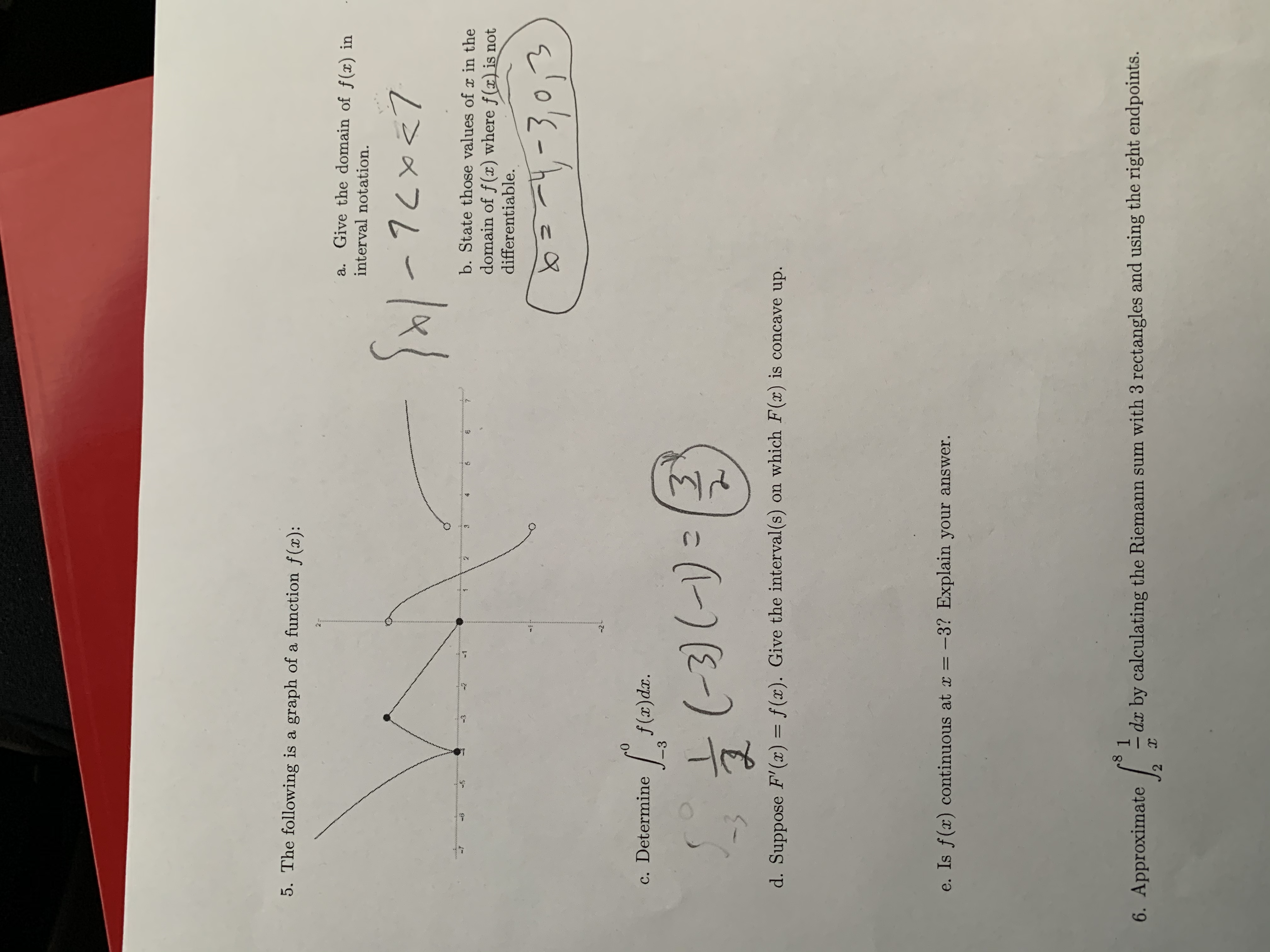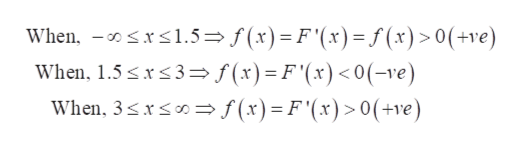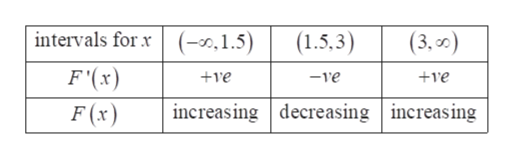# 5. The following is a graph of a function f(x)a. Give the domain of f(x) ininterval notation.b. State those values of r in the-3-12domain of f(x) where f(x)is notdifferentiable.ef(a)dar.c. Determine-33) (-)-3d. Suppose F'(x) = f(x). Give the interval(s) on which F(x) is concave up.e. Is f(x) continuous at x-3? Explain your answer.81dx by calculating the Riemann sum with 3 rectangles and using the right endpoints.6. ApproximateC)

Question
56 viewshelp_outlineImage Transcriptionclose5. The following is a graph of a function f(x) a. Give the domain of f(x) in interval notation. b. State those values of r in the -3 -1 2 domain of f(x) where f(x)is not differentiable. ef(a)dar. c. Determine -3 3) (-) -3 d. Suppose F'(x) = f(x). Give the interval(s) on which F(x) is concave up. e. Is f(x) continuous at x -3? Explain your answer. 8 1 dx by calculating the Riemann sum with 3 rectangles and using the right endpoints. 6. Approximate C) fullscreen
check_circle

Step 1

(d) It is given that F '(x) = f(x). Both F(x) and f(x) are different functions.

Value of F '(x) is known from the graph so, find out the interval where F '(x) is positive and negative.help_outlineImage Transcriptionclose<1.5 f(x)= F'(x)=f(x)>0(+ve) 3> f(x)= F'(x) < 0(-ve) When, When, 1.5 When, 3x = f(x)=F'(x)>0(+ve) fullscreen
Step 2

Now, create the table for different intervals for F(x) and F '(x).help_outlineImage Transcriptioncloseintervals for x (-0,1.5) (3,d) (1.5,3) F'(x) ve +ve -ve increasing decreasing increasing F (x) fullscreen
Step 3

Then, as per the table F(x) is increasing in x ? (-∞, 1.5) and decreasing in x ? (1.5, 3).

Therefore, x = 1.5 is the point of maximum and concavity is downward.

Again, F(x) is decreasing in x ? (1.5, 3) and...

### Want to see the full answer?

See Solution

#### Want to see this answer and more?

Solutions are written by subject experts who are available 24/7. Questions are typically answered within 1 hour.*

See Solution
*Response times may vary by subject and question.
Tagged in

### Functions